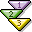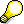Collecting Data for a Cost Object HierarchyUse

When you execute a data collection run for a cost object hierarchy, the values of orders assigned to the cost object hierarchy are rolled up. The system generates a summarization object for every cost object node. A summarization object contains the values that were added together corresponding to the original period and cost element.

The following data that was posted to a cost object node or an order assigned to a cost object node can be summarized:

• Planned values
• Actual values
• Target values
• Work in Process
• Variances
• Plan/actual quantitiesThe system can summarize only the entire cost object hierarchy, but not a subhierarchy (as is the case with summarization hierarchies).

Prerequisites

It is recommended that you execute the data collection run after each period-end closing, thus summarizing current data such as target costs, work in process and variances.

If you want to monitor actual costs, it can also be helpful to execute a data collection run during a period before period-end closing activities.

Procedure

To summarize the data for a cost object hierarchy:

1. Choose Accounting ® Controlling ® Product Cost Controlling ® Cost Object Controlling ® Product Cost by Period ® Information System ® Tools ® Data Collection ® Cost Object Hierarchy.
2. The screen Cost Object Summarization appears.

3. Enter the following data:
• Cost object (must be flagged as the top of the hierarchy)
• From period/fiscal year and to period/fiscal year

In the summarization run the values for the periods within the specified summarization time frame are reset and recalculated. Data on the summarization object for periods outside of the summarization time frame is retained. This prevents the data calculated in an earlier summarization run from being deleted when the orders are reorganized.

1. In the standard system, the indicator Background processing is turned on. If you want to perform the summarization run online, you must turn off this indicator.
2. Choose Execute.

If you have turned off the indicator for background processing, a dialog box appears in which you must confirm the summarization run.

An overview screen is now displayed that shows the nodes of the hierarchy processed. The different levels of the hierarchy and the designations (such as the designation of the cost object ID and the material number) are shown.

You can use the following functions:

• Message log

The message log lists any errors that occurred and any information messages that were issued during summarization.

• Table statistics

The table statistics specify how many totals records were written during summarization.